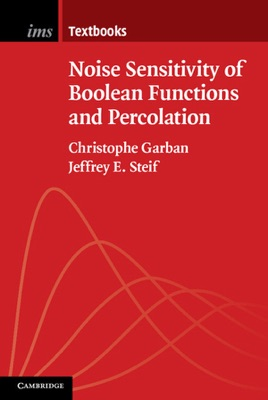• 18,99 €

## Descrizione dell’editore

This is a graduate-level introduction to the theory of Boolean functions, an exciting area lying on the border of probability theory, discrete mathematics, analysis, and theoretical computer science. Certain functions are highly sensitive to noise; this can be seen via Fourier analysis on the hypercube. The key model analyzed in depth is critical percolation on the hexagonal lattice. For this model, the critical exponents, previously determined using the now-famous Schramm–Loewner evolution, appear here in the study of sensitivity behavior. Even for this relatively simple model, beyond the Fourier-analytic set-up, there are three crucially important but distinct approaches: hypercontractivity of operators, connections to randomized algorithms, and viewing the spectrum as a random Cantor set. This book assumes a basic background in probability theory and integration theory. Each chapter ends with exercises, some straightforward, some challenging.